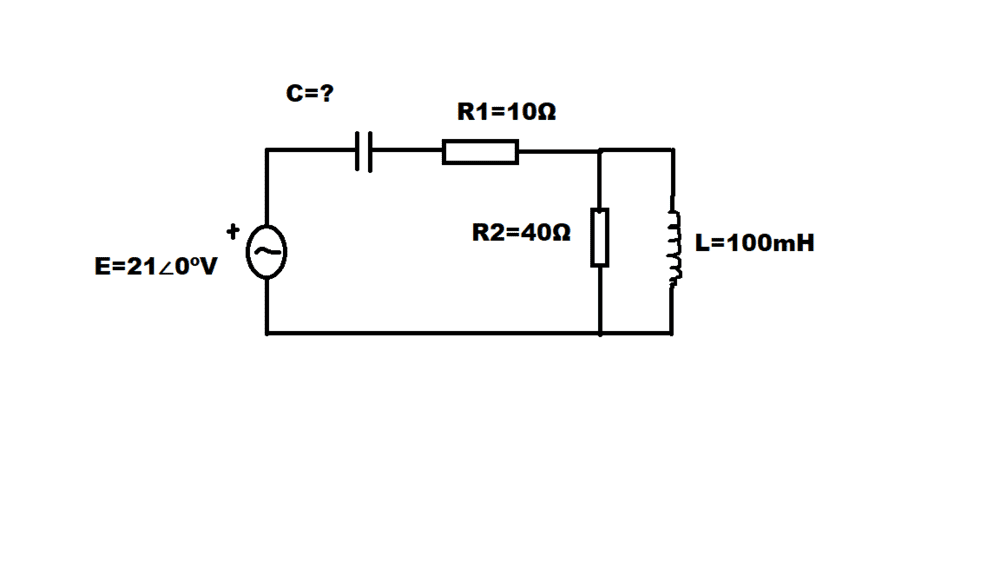# Determine the capacitor value so that this circuit is in resonance

Homework Statement:
Need to determine capacitor value so that the circuit is in resonance
Relevant Equations:
E=21∠0º
ω=10^3 rad/s
R1=10Ω
R2=40Ω
L=100mh
C=?L=100mh=0.1H
ω=10^3 rad/s -> f = 159Hz
XL= ωL= 2πfL= 2π*159*0.1= 99.90 Ω
Z parallel = [(XL∠90º)*R2] / [(XL∠90º)-R2]= 37.13∠-21.8º
XC= 1/ωC= 1/(2 π f C)
I dont see how I am supposed to get to C

Last edited:

## Answers and Replies

TSny
Homework Helper
Gold Member
L=100mh=0.1H
ω=10^3 rad/s -> f = 159Hz
XL= ωL= 2πfL= 2π*159*0.1= 99.90 Ω
OK. Here, it is easier to use XL = ωL rather than XL = 2πfL. Your value of 99.90 has a bit of round off error.
Z parallel = [(XL∠90º)*R2] / [(XL∠90º)-R2]
Check the sign of R2 in the denominator.

Are you familiar with using complex numbers to represent impedances? For me, that makes things easier.
I dont see how I am supposed to get to C
Think about how to combine the resistance R1 and the reactance of C with the result for Z_parallel. Can you see that C, R1, and Z_parallel are all in series?

•baywa2
Ok
so Z parallel = [(XL∠90º)*R2] / [(XL∠90º)+R2]
I get Z is some 37 Ω
So do I then use R1 and C for Z? Z=R1+XC∠-90º
Then add the Z and Zpar? 10+37+XC∠-90º -> 47+XC∠-90º

TSny
Homework Helper
Gold Member
Ok
so Z parallel = [(XL∠90º)*R2] / [(XL∠90º)+R2]
I get Z is some 37 Ω
There should also be a phase angle for the 37 Ω.

So do I then use R1 and C for Z? Z=R1+XC∠-90º
Then add the Z and Zpar? 10+37+XC∠-90º -> 47+XC∠-90º
You can't combine the 10 and the 37 to get 47 since the 37 has a nonzero phase angle. It's like adding vectors. The 10 Ω corresponds to a vector pointing along the x axis since it has zero phase angle. The 37 Ω corresponds to a vector pointing at some angle above the x axis.